Critical Voltage Proximity Index Investigation For Transmission Systems | OMICS International
Global Journal of Technology and Optimization
All submissions of the EM system will be redirected to Online Manuscript Submission System. Authors are requested to submit articles directly to Online Manuscript Submission System of respective journal.

# Critical Voltage Proximity Index Investigation For Transmission Systems

Alexis Polycarpou*, Hassan Nouri, Dan Micu

Frederick University, University of the West of England, Cluj-Napoca University

*Corresponding Author:
Alexis Polycarpou
Frederick University
University of the West of England
Cluj-Napoca University
E-mail: [email protected]rederick.ac.cy, [email protected], [email protected]

Received date: February 2012, Revised date: April 2012, Accepted date: May 2012

Visit for more related articles at Global Journal of Technology and Optimization

#### Abstract

A developed mathematical critical voltage proximity index is investigated in this paper. Mathematical description of the index is provided. Background theory on established statistical indices, as well as mathematical indices recently developed, are presented, highlighting the successful application of the index in distribution systems, featuring low X/R ratio values, and the need for investigation of the index at transmission level. The index is applied on a transmission test system and verified for its compatibility through the use of tap changing transformers as voltage sag mitigation scheme. Three induction motor load variation scenarios are investigated in order to establish the effectiveness and accuracy of the index. The development of a multiple tap changing controller scheme within PSCAD software is also described. The index improves the power quality of a system, through providing a tool for on-line voltage sag mitigation, featuring multiple potential applications.

#### Keywords

Voltage Sag index, Induction Motors, Tap Changing, X/R ratio, Critical Voltage Levels.

#### Introduction

Voltage sag is mostly a distribution issue. Most of the customers are supplied by it, and it is where the effect of a voltage sag is greater. In transmission systems, a combination of increased current flow and long transmission lines cause the voltage drop along the line to be of significant value. Thus the voltage is boosted up to acceptable levels through techniques established at substation locations.

The interest in voltage dips has been increasing rapidly in the last decade, due to problems induced on several types of equipment, such as adjustable speed drives, process control equipment and computers [1, 2]. Tripping can have devastating effects on the production and thus the financial condition of the associated plant . Available voltage sag indices use a statistical approach to characterize the events from the power quality point of view, thus identifying where there is need for improvement in the system [4-13]. These Indices are presented in the Literature review section. The voltage sag index investigated in this paper is of mathematical rather than statistical nature. The mathematical development of the index is in effect a result of merging mathematical voltage sag indices previously developed and verified for various transmission and distribution line X/R ratio values as well as loading conditions [14-19]. Detailed reference to those voltage sag indices is presented in section 2.2.

In this paper, voltage dips are caused by introduction of loads at the end of the feeder. The loads consist of induction motor groups [20, 21].Each of these groups consists of six induction motors all of these rated at 2 MW, 3.355 MVA. The influence of induction motor loads on the characterization of voltage sags is of great importance, as indicated by researchers [20-24]. During starting the motor will accelerate, drawing a high reactive current from the supply, until the steady-state speed is reached, causing prolonged post fault voltage sag . The deceleration and acceleration of motors influences the duration and shape of voltage sags. If a motor load is wired for on-line direct starting, even in the absence of fault in the system, the high reactive starting current will cause a voltage sag on the supply feeder. The voltage drop along the feeder increases as more current flows through its resistive and inductive elements. Thus the receiving end voltage reduces. The problem arises since consumers supplied by the same feeder might have equipment sensitive to voltage variations. The reduction caused by the newly introduced load could affect all other consumers.

Since the impact of the dip depends on the associated equipment sensitivity of the adjacent consumers, the need arises for an online critical voltage proximity index.

The index should be able to be implemented in conjunction with various available mitigation techniques. For this investigation tap-changing transformers are used as a mitigation solution. The purpose of the paper is not to focus on a particular mitigation technique but to illustrate the applicability and effectiveness of the mathematical index.

An electronic tap changer is a device with thyristor-based switches fitted to power transformers for regulation of the output voltage to required levels. This is normally achieved by changing the ratios of the transformers on the system by altering the number of turns in one winding of the appropriate transformer/s. Supply authorities are under obligation to their customers to maintain the supply voltage between certain limits. Tap changers offer variable control to keep the supply voltage within these limits.

Tap changing transformers are used to illustrate the compatibility time and magnitude discriminative characteristics, effectiveness, and fast response of the index. As a real time calculation index, those are the characteristics the critical value proximity index is required to possess.

It should be noted that in practice the principle of voltage sag mitigation through the use of tap changing transformers is not feasible as it is restricted by time constrains related to switching procedures. Voltage sags are pronominally a transient event and thus it is important to illustrate that due to its fast response, the index can be used for such events.

Literature Review

The Critical Voltage Proximity Index is based on mathematical voltage sag indices. The mathematical indices were not developed in order to replace the statistical ones, but to complement them. In the following parts of this section statistical and mathematical voltage sag indices are presented.

Theory on Statistical Voltage sag Indices

Any available statistical voltage sag index can be classified within the following three categories [2,3].

Single-event index: a parameter indicating the severity of a voltage or current event, or otherwise describing the event. Each type of event has specific single-event indices.

Single-site index: a parameter indicating the voltage or current quality or a certain aspect of voltage or current quality at a specific site.

System index: a parameter indicating the voltage or current quality or a certain aspect of voltage or current quality for a whole or part of a power system.

The procedure to evaluate the power systems performance regarding voltage sags is as follows.

Step 1- Obtain sampled voltages with a certain sampling rate and resolution.

Step 2- Calculate event characteristics as a function of time, from the sampled voltages.

Step 3- Calculate single event indices from the event characteristics.

Step 4- Calculate site indices from the single-event indices of all events measured during a certain period of time.

Step 5- Calculate system indices from the site indices for all sites within a certain power system.

Each step mentioned above will be briefly discussed in the following parts of this paper, with more weight given on the steps involving the various index types.

Step 1 will not be discussed at all since it simply represents the procedure to obtain voltage samples for every event with a certain sampling rate and resolution.

Event Characteristics

From the sampled voltages, the characteristic voltage magnitude as a function of time can be obtained. To accomplish that three methods are presented use three phase measurements .

Method of Symmetrical components: From the voltage magnitude and phase angle, a sag type is obtained along with the characteristic voltage, the PN factor and Zero sequence voltage. The characteristic magnitude (the absolute value of the characteristic complex voltage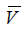) can be used to characterize three-phase unbalanced dips without loss of essential information. Using characteristic magnitude and duration for three-phase unbalanced dips, corresponds to the existing classification (through magnitude and duration) for singlephase equipment.

Method based on six rms voltages. The procedure used in this method is to calculate the zero sequence component of the voltage and remove it from the phase voltages. The new phase to phase voltages can then be calculated. From the phase to phase voltages and the three phase voltages, the rms values can be calculated. The characteristic magnitude would then be the lowest of the six rms voltages.

Single Event Indices

From the event characteristics as a function of time, a number of indices are determined that describe the event.

For some applications the phase angle at which the sag begins is important and called ‘point on wave of dip initiation’. Using its algorithm, the exact beginning of the voltage dip can be identified, .

In addition the point on wave of voltage recovery allows the precise calculation of the sag duration.

The maximum phase shift can be obtained from the voltage characteristic versus time and can be used for accurate phaseangle jump calculation.

The maximum slew rate, zero sequence voltage and minimum PN factor are mentioned as potential single event indices.

The Canadian electrical association uses two approved quality indices. Rms Overvoltage and Under voltage (RMSO and RMSU) 

The indices are assessed over intervals equal to the time needed for equipment to reach its steady-state temperature. The heating time constant, which varies with the size and nature of the equipment, has been divided into three classes; Highly sensitive electronic systems with a heating time constant of less than 600 ms, power electronics with a 2-min heating time constant, and electrical apparatus with a heating time constant exceeding 24 min.

Canadian utilities have retained 2-min heating time constant to assess the factor related to over voltages and under voltages. This factor is measured over 10-min intervals (5×2 min).

The most-commonly used single event indices for voltage dips are ''retained voltage'' and ''duration''. It is recommended to only use the rms voltage as a function of time, or the magnitude of the characteristic voltage for three-phase measurements, to calculate the duration.

Using the characteristic voltage magnitude versus time, obtained in Step 2, the retained voltage and duration can be obtained as follows.

The basic measurement of a voltage dip and swell is Urms(1/2) on each measurement channel. Where Urms(1/2) is defined as the value of the rms voltage measured over one cycle and refreshed each half cycle .

For single-phase measurements:

A voltage sag begins when the Urms(1/2) voltage falls below the dip threshold, and ends when the Urms(1/2) voltage is equal to or above the dip threshold plus the hysterisis voltage.

The retained voltage is the smallest Urms(1/2) value measured during the dip. The duration of a voltage dip is the time difference between the beginning and the end. Voltage sags may not be rectangular. Thus, for a given voltage sag, the duration is dependent on a predefined threshold sag value.

The user can define the sag threshold value either as a percentage of the nominal, rated voltage, or as a percentage of pre-event voltage. For measurements close to equipment terminals and at distribution voltage levels, it is recommended to use the nominal value. At transmission voltages, the preevent voltage may be used as a reference.

The choice of threshold obviously affects the retained voltage in percent or per unit. The choice of threshold may also affect the measurement of the duration for voltage dips with a slow recovery. These events occur due to motor starting, transformer energizing, post-fault motor recovery and post-fault transformer saturation.

For a multi channel three phase measurement:

The voltage sag starts when the rms voltage Urms(1/2), drops below the threshold in at least one of the channels, and ends when the rms voltage recovers above the threshold in all channels. The retained voltage for multi-channel measurement is the lowest rms voltage in any of the channels.

Several methods have been investigated leading to a single index for each event. Although this leads to higher loss of information, it simplifies the comparison of events, sites and systems. The general drawback of any single-index method is that the result no longer directly relates to equipment behavior. Single indices often used are Loss of Voltage, Loss of Energy, method proposed by Thallam [6,10], and the method proposed by Heydt .

Site Indices

Usually the site indices have as inputs the retained voltage and duration of all the sags recorded at a site over a given period. Available RMS Variation Indices for Single Sites are described below.

The SAFRI index (System Average RMS Variation Frequency Index) relates how often the magnitude of voltage sag is below a specified threshold. It is a power quality index which provides a rate of incidents, in this case voltage sags, for a system, .

SARFI-X corresponds to a count or rate of voltage sags, swell and interruptions below a voltage t threshold. It is used to assess short duration rms variation events only.

SARFI-Curve corresponds to a rate of voltage sags below an equipment compatibility curve. For example SARFI-CBEMA considers voltage sags and interruptions that are not within the compatible region of the CBEMA curve. Since curves like CBEMA do not limit the duration of a rms variation event to 60 seconds, the SARFI-CBEMA curve is valid for events with a duration greater than ½ cycle. Other curves used are the ITIC Curve Scatter Plot, SEMI Curve Scatter Plot, and the Voltage sag co-ordination chart-IEEEStd.493 and 1346.

Calculation methods for site indices often used are

Method used by Detroit Edison, and methods proposed by Thallam [6,9,10].

Non-rectangular events are events in which the voltage magnitude varies significantly during the event. IEEE Std.493- 1997 describes a method to include non-rectangular events in the voltage-sag coordination chart. Alternatively, the function value can be defined as the number of times per year that the rms voltage is less than the given magnitude for longer than the given duration.

Each authority (e.g. EPRI/Electrotek, Canadian electrical association, ESKOM) seems to have its own standards for calculating the various limit violations.

System Indices

System indices are typically a weighted average of the singlesite indices obtained for all or a number of sites within the system.

The difficulty is in the determination of the weighting factors. In order to assess any indices for the system, first monitoring of the quality of supply must take place. When the EPRI-DPQ power quality monitoring program placed monitoring equipment on one hundred feeders, these feeders needed to adequately represent the range of characteristics seen on distribution systems. This required the researchers to use a controlled selection process to ensure that both common and uncommon characteristics of the national distribution systems were well represented in the study sample. Thus a level of randomness is required.

Many devices are susceptible to only the magnitude of the variation. Others are susceptible to the combination of magnitude and duration

One consideration in establishing a voltage sag index is that the less expensive a measuring device is, the more likely it will be applied at many locations, more completely representing the voltage quality electricity users are experiencing.

With this consideration in mind, sag monitoring devices generally fall into two classes, Hansen :

Less expensive devices that can monitor the gross limits of the voltage sag, and more expensive devices that can sample finer detail such as the voltage-time area and other features that more fully characterize the sag.

The sag limit device senses the depth, of the voltage sag. The sag area device can sample the sag in sufficient detail to plot the time profile of the sag. With this detail it could give a much more accurate picture of the total sag area, in volt-seconds, as well as the gross limits. The retained voltage, Vr, is also shown.

The developed rms variation indices proposed by electrotek (EPRI), are designed to aid in the assessment of service quality for a specified circuit area. The indices are defined such that they may be applied to systems of varying size. Values can be calculated for various parts of the distribution system and compared to values calculated for the entire system. Indices capable to assess rms magnitude variation and the combination of magnitude and duration are

System Average RMS (Variation) Frequency Indexvortage (SARFIx), System Instantaneous Average RMS (Variation) frequency Indexvortage (SIARFIx), System Momentary Average RMS (Variation) Frequency Indexvortage (SMARFIx), and System Temporary Average RMS (Variation) Frequency Indexvortage (STARFIx).

Mathematical Voltage sag Indices

In this section of the paper detailed mathematical description, theoretical background and verification results of developed voltage sag indices are presented.

Any feeder can be modeled by an equivalent two-port network, which is represented by the following equations.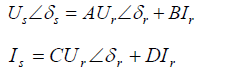(1)

Where: Us - Sending end voltage, Is -Sending end current, Ur - Receiving end voltage, Ir - Receiving end current, δs – Sending end voltage angle, δr- receiving end voltage angle, A,B,C,D

Two port network constants.

For a short length line the two port network parameters can be approximated as: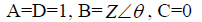(2)

Where:

Z=Transmission line impedance

θ= Transmission line impedance angle

Rearranging (1) and substituting the A, B , C, D parameters in the result, (3) is obtained.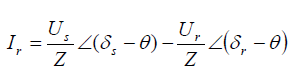(3)

Substituting Ir from (3) in equation (1)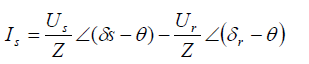(4)

Equations (5) and (6) represent the apparent power at the sending and receiving end respectively.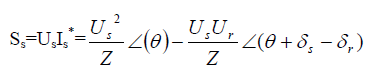(5)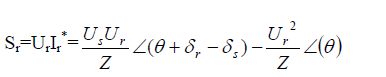(6)

The active and reactive power at the sending and receiving end of the transmission line can be obtained from the real and imaginary parts of equations (5) and (6) .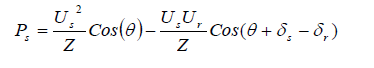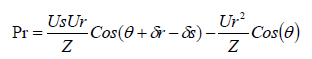(7)

Substituting Ur=ζUs, in the receiving Power equation (7) Where ζ signifies the voltage magnitude during the sag as per unit function of the sending voltage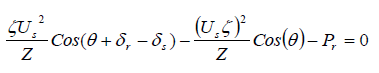(8)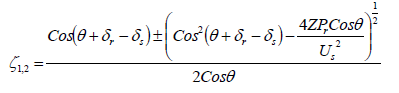(9)

Equation (9) provides an index for voltage sag calculation, as a per unit value of the sending end voltage, through angles and power demand. Various investigations have been carried out towards the validation of the index. The concept and results of which are presented below.

The quadratic voltage sag index equation, since it is obtained through a quadratic equation, has two solutions.

Thus ζ1 will be valid for a specific range of parameters. In the same way ζ2 will be valid for a different range of parameters. In theory equatio

In theory equation (9) can be described graphically by the graph in Figure 1.

The point of intersection of ζ1 theoretical and ζ2 theoretical, occurs when (ζ 12 )cosθ is equal to zero, when both solutions are identical.

Investigation has been carried out to establish the range of each solution with variation of the feeder line X/R ratio for various loadings [14,15]. The investigations resulted to a clear definition of the choice of the quadratic solutions. The relationship between the slope of ζ1 and ζ2 with the index accuracy and choice of solution is described by (10)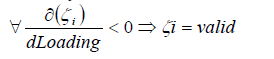(10)

Where i could have the value 1 or 2 corresponding to the solution

Voltage sag index ‘ξ’

The index ξ is also based on the power flow equations. Substituting Ur = ζUs in the sending Power equation (7), equation (11) yields, where ξ signifies the voltage magnitude during the sag as per unit function of the sending voltage.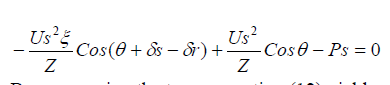(11)

By rearranging the terms equation (12) yields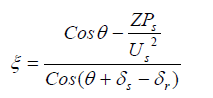(12)

ξ represents the calculated voltage at the receiving end for a line with input voltage 1 pu. If the line input is not voltage regulated the receiving voltage can be calculated by multiplying the sending end voltage with the index variable.

The index was investigated for various loading levels with the use of speed controlled and torque controlled motor loads. Accuracy limits were defined with regards to loading .

Combined Voltage Index (CVI)

The result of the investigation of the ξ and ζ indices led to the definition and investigation of a combined voltage sag index (CVI) [16-18].

Combining (9) and (12), an equation representing the Combined Voltage Index (CVI) yields.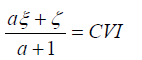(13)

The ‘a’ parameter signifies the scaling factor  and is defined as :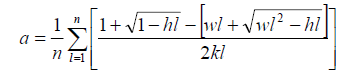(14)

Where: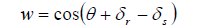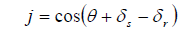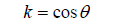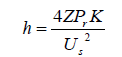The value of the scaling factor for distribution systems was calculated to be in the range of 1.46 to 2.18. In an effort to make the index less dependent on variables, a value of 1.6 was chosen to be allocated to the scaling factor for all distribution system cases.

Equations representing ζ, ξ and CVI provide a tool to calculate the load voltage, as a per unit value of the sending end voltage. The equations are functions of receiving end variables such as the the receiving end voltage angle, δr, and the receiving end power Pr. The receiving end power can be described by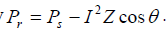A mathematical description of δr is provided by equation (15) .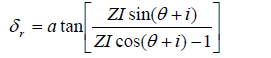(15)

Using the power and load angle mathematical descriptions, the index consists of only single point quantities.

The CVI index accuracy was investigated and verified for a number of X/R ratios corresponding to transmission and distribution system lines [16,17,24]. Various motor power factors and loading levels were also used in order to establish the behaviour of the index The index accuracy deviation is small for the investigated distribution cases. An increase of X/R ratio, associated with transmission network lines, causes an increase of index accuracy deviation . Thus it is required to carry out an investigation regarding the performance of the critical voltage proximity index (CVPI), which also is a function of ξ and ζ, with regards to transmission systems

Critical Voltage Proximity index theory

In this section of the paper the mathematical formulation of the CVPI as well as the methodology followed towards the investigation is presented.

CVPI mathematical formulation

Using equations (9) and (12) the Critical Value Proximity Index (CVPI) shown in equation (16) is derived.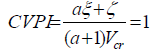(16)

‘Vcr’ in equation (16) represents the critical threshold value at which the equation will converge to one. The value of the index is greater than one if the voltage of the line the index is describing is higher than the critical value. The critical value can be chosen according to the customer’s sensitivity to voltage magnitude.

Investigation Methodology

The index has been verified with regards to the application in distribution systems. . A controller was designed to use the mathematical index output in order to control the tap changer setting of a distribution transformer. When the voltage was below a certain value defined as critical voltage, the controller forced the tap changer to step up the secondary winding voltage to reduce the sag.

For transmission system applications, the index was partly investigated in [25, 26].

As index accuracy deviation increases with an increase of X/R ratio of the line, a more detailed investigation is required to be carried out. The investigation presented in this paper, for transmission system index applications.

Investigation Scenarios

The transmission system provides a path for the power to flow from the generators to the distribution system. In this section the applicability of the critical voltage proximity index, with the use of tap changing transformers, in transmission is investigated.

The principle of mitigation through tap changing used in this paper at transmission level is that increasing the voltage along the line reduces the current magnitude and thus reduces the voltage drop. In order to illustrate the operation of the mitigation technique, the system in Figure 2 can be considered.

Observing Figure 2, in order to step up the voltage during high current flow, tap changers are required at both ends of the line.

Motor Groups are used in the simulation to represent heavy loads connected to the distribution system. These loads are being switched into the system one to represent minimum load by one motor group, and Maximum load by five groups. Induction motors are chosen due to their high current demand and contribution towards voltage sag.

When the voltage drops below a defined value during transient or steady state the tap changer steps up the voltage to reduce losses, according to the controller operation. The maximum increment of the tap depends on the characteristics of the transformer. In this paper for demonstration reasons it is chosen to use a maximum tap of 25%, using five incremental steps of 5%. The simulation is performed for 10 seconds with load addition at 1 2 4 6 and 8 seconds.

In order to illustrate the effectiveness of the method and the controller, three different loading scenarios are used for transmission as well as for distribution. Figure 3 shows the three loading scenarios investigated.

The load variation cases are chosen to be of that shape in order to fully investigate the performance of the index. The results obtained from the three loading variation cases for the transmission system are presented in the Results section of this paper.

Controller modelling

A change in the transformer tap setting, within the PSCAD environment, is modeled as a change in the turns-ratio of the transformer. When the tap changer is enabled, an input appears which controls the tap setting. An on-line tap input of 1.0 corresponds to a 100% tap i.e. no tap adjustment. The PSCAD help module recommends changing tap settings in steps, either through manual adjustment or from a controller with appropriate delays and steps built in. In this case a controller is constructed to accommodate the nature of the index shown in (16). The controller is shown in Figure 4.

As mentioned previously, the output of the index is a value that reduces according to voltage reduction and reaches the value of ‘one’ when the critical voltage value has been reached. In order to have five taps it is necessary to define five critical voltage values. In this case the values are chosen to be 0.98, 0.94, 0.91, 0.88, and 0.85. These values of critical voltage can be seen in Figure 4 as Vcr1 to Vcr5. The value of the index is calculated on-line. The point of critical voltage is reached when the value of CVPI is equal to one or when 1-CVPI reaches 0. That allows the single input level comparator to be used in the controller. When the value of zero is reached, after user specified delay, the signal will enable a two-input selector to change the value of the tap. This procedure repeats itself when the next critical voltage has been reached. In case of load shedding, the controller has the ability to recognise overvoltages as well and it will automatically switch to the most appropriate tap setting .

At the start of the simulation, half second is allowed for system initialization before applying the tap changer controller.

The user specified delay allows the scheme to discriminate sags in the system according to their duration, or sags which will affect the customer and sags which will not. That value is defined according to the sensitivity of the equipment supplied by that feeder, thus effectively protects the customers. The delay value can also be adjusted according to the capability of the mitigation equipment used and its switching speed. The fastest response time for an electronic tap changer using thyristor-based switches for example, is one and a half cycles . In the cases under investigation the delay is set to zero, and the controller forces a tap change for every critical limit violation.

Results and Discussion

In this section the results of the investigation of the three case scenarios are presented and discussed.

The Load variation curve corresponding to this case, as seen in Figure 4, represents a steady increase in load power demand. Figure 5 illustrates the voltage profile for the equivalent power system demand.

As expected, the voltage drop towards the load bus increases with the increase of load demand. However the effect of load increment would have been worse without the operation of the transformer tap. Figure 6 illustrates the tap value for the duration of the simulation corresponding to this case of load variation.

Observing Figures 5 and 6 it can be seen that when the voltage dropped below a critical value, the tap value changed to the corresponding preset level. Thus during this test case, the index, the controller and the whole scheme is operating as required.

The Load variation curve in this case, as seen in Figure 3, represents a heavy increase in load power demand at one second corresponding to four loads. The fifth is switched in the system at two seconds, followed by a reduction of load in steps of two, one, and one motor at four, six and eight seconds respectively. Figure 7 illustrates the voltage profile for the equivalent power system demand.

It can be seen from Figure 7 that the receiving end voltage follows the expected profile for the loading under consideration. The effect on the tap setting of the transformer can be seen in Figure 8.

As previously the tap controller switches the value of the tap setting according to which value of critical voltage has been reached. The above statement was verified through detail investigation of the switching time steps.

Observing Figure 7 and 8 it can be seen that the transient switching of four loads at one second forces the tap to go to its maximum value (1.250) before settling down at 1.150. That effect is due to the magnitude and duration of the voltage sag the load addition has caused. After the load variation at 4 seconds, the voltage reaches steady state just over the critical voltage which corresponds to the tap changer setting of 1.2. Momentarily the controller forces the tap to that value before reaching steady state at 1.15. The index proposed can discriminate in terms of both magnitude and duration and thus can tackle specific disturbances, which cause low power quality ratings.

The Load variation curve corresponding to this case, as seen in Figure 3, represents a heavy increase in load power demand at one second corresponding to all five loads. Load reduction follows, one load every switching time. Figure 9 illustrates the voltage profile for the equivalent power system demand.

The equivalent tap changer plot can be seen in Figure 10.

Figure 10 completes the illustration of curves regarding the Transmission system. It has been shown that for this type of system, and corresponding feeder X/R ratio, the mitigation technique is effective and discriminative.

Thus it can be concluded that the index is applicable for voltage sag mitigation in cases of transmission line X/R ratios. It must be noted that in order to implement this scheme within PSCAD environment, a change of base for some parameters used by the controller is required for the Distribution system. This change is due to the voltage change of base on the load bus according to the transformer tap changer setting.

The index has proved to be effective and accurate for all three test cases.

Future Research Directions

Having established the applicability of the index at distribution as well as transmission level, other mitigation techniques, applicable on power systems, can be investigated for their speed and accuracy in conjunction with the index. Such techniques can be Solid State Fault Current Limiters, Series voltage restoration techniques, active series voltage injection; Series shunt capacitors, Advanced Static Var Compensators, dynamic voltage restorers, etc.

Practical implementation of the technique on real test system is also included in the future research directions, with the possibility of theoretical optimization techniques applied on the indices equations to provide the ideal equation parameters for voltage sag reduction.

#### Conclusions

A developed Critical Voltage Proximity Index (CVPI) investigation is presented in this paper. Previous investigations of mathematical indices resulted in negligible accuracy deviations for distribution X/R ratios. Thus the index investigation presented is carried out for transmission systems featuring high X/R ratio values. A tap changing transformer scheme is used to illustrate the effectiveness and applicability of the index. For that purpose, a controller was successfully designed and implemented in PSCAD. The (CVPI) implemented with the controller provides the user with the ability to define when the mitigation technique is enabled in terms of voltage sag magnitude and duration, making the index highly discriminative and widely applicable. The index is successfully applied to transmission systems, for three cases of loading variations. The index is adaptable to incorporate future changes in system parameters, and could be applicable with other available mitigation techniques. According to the findings of this paper the index was proven to be fast, effective and discriminative, and can regulate and improve the power quality of a system.

#### References

Select your language of interest to view the total content in your interested language

### Article Usage

• Total views: 12053
• [From(publication date):
December-2012 - Nov 19, 2019]
• Breakdown by view type
• HTML page views : 8267Can't read the image? click here to refresh